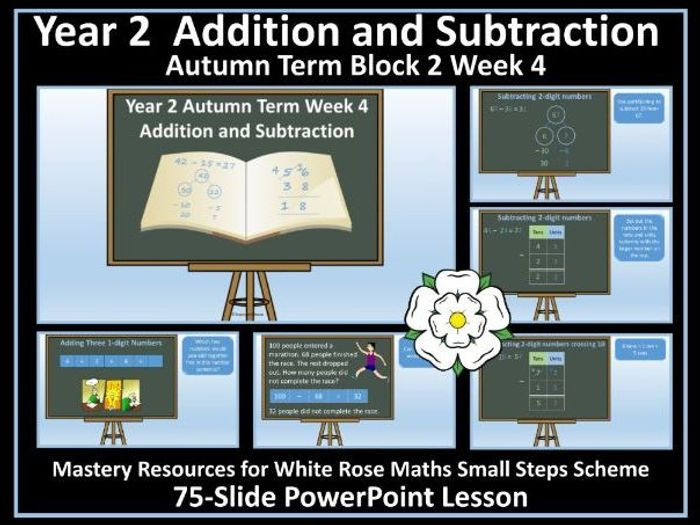This resource contains a fully editable, 80-slide PowerPoint lesson to support the delivery of the Year 2 Addition and Subtraction - Autumn Term - Block 2 White Rose (WRM) small steps programme. This PowerPoint is for Week 4.

The PowerPoint contains tasks and activities (pictorial and abstract) linked to the White Rose small steps with an emphasis on the mastery approach to learning advocated by the White Rose scheme and includes reasoning and problem-solving tasks. It can be used flexibly - for whole lessons, with small groups, as a starter, for consolidation and for revision.

The PowerPoint focuses on the following objectives and small steps:

Year 2 Maths’ National Curriculum Objectives

Recall and use addition and subtraction facts to 20 fluently, and derive and use related facts up to 100.

Add and subtract numbers using concrete objects, pictorial representations, and mentally, including: a two-digit number and ones; a two-digit number and tens; two two-digit numbers; adding three one-digit numbers.

Show that the addition of two numbers can be done in any order (commutative).

Solve problems with addition and subtraction: using concrete objects and pictorial representations, including those involving numbers, quantities and measures; applying their increasing knowledge of mental and written methods.

Recognise and use the inverse relationship between addition and subtraction and use this to check calculations and solve missing number problems.

White Rose Small Steps

Subtract a 2-digit number from a 2-digit number – not crossing ten
Subtract a 2-digit number from a 2-digit number – crossing ten - subtract ones and tens
Bonds to 100 (tens and ones
Add three 1-digit numbers

You may also be interested in an accompanying 21-page workbook and progress check which can be used alongside the PowerPoint.

Year 2 Week 1 Addition and Subtraction: Autumn Term Block 2 Workbook 4

More Maths Resources

Thinking of publishing your own resources? Check out this step-by-step guide:

How to Become a Successful TES Author: Step by Step Guide

\$3.91
Save for later

Info

Created: Sep 18, 2018

Updated: Oct 21, 2019

pdf, 322 KB

p01

pdf, 440 KB

p02

pdf, 470 KB

p03

Report a problem

Tes Paid Licence

How can I re-use this?